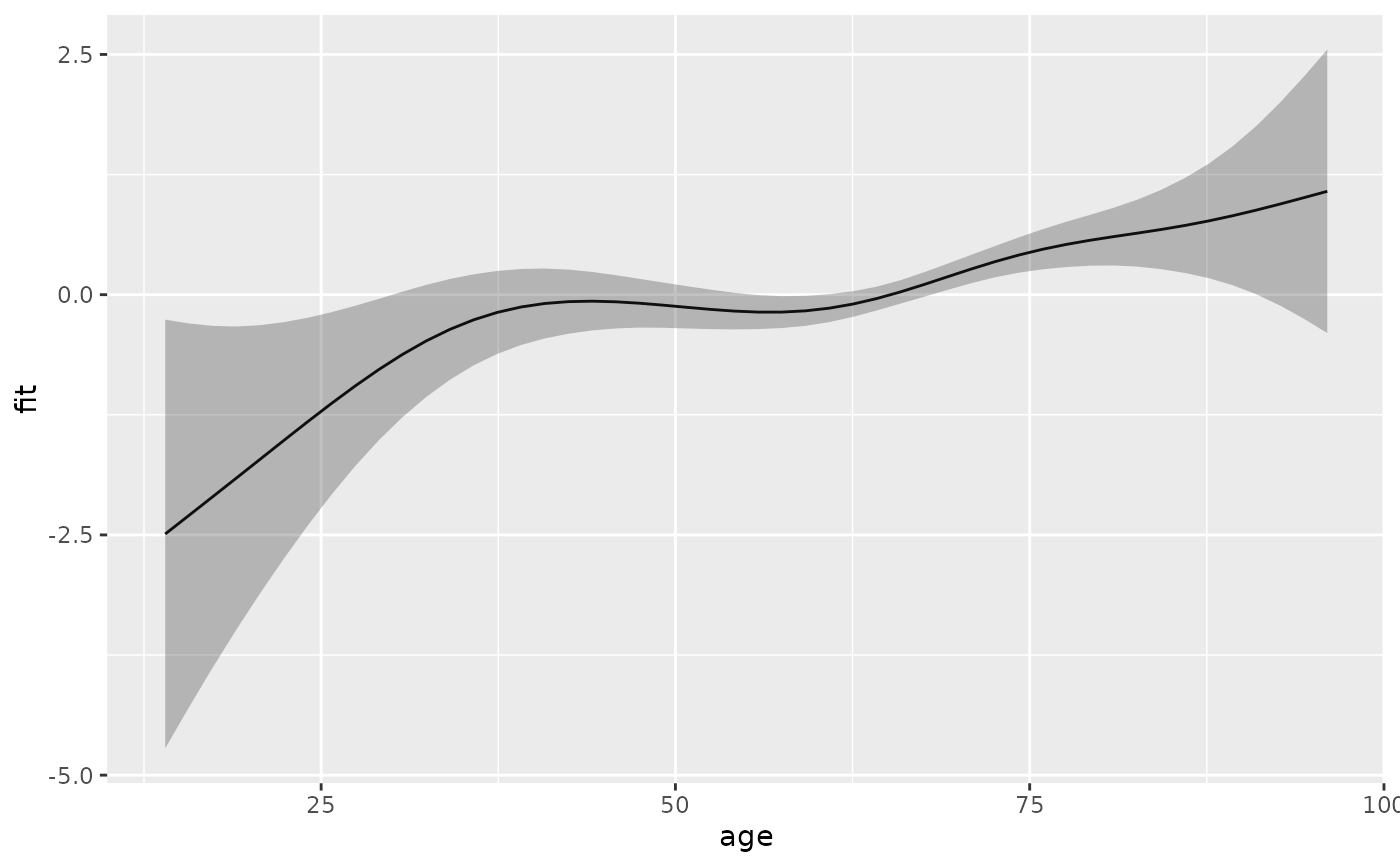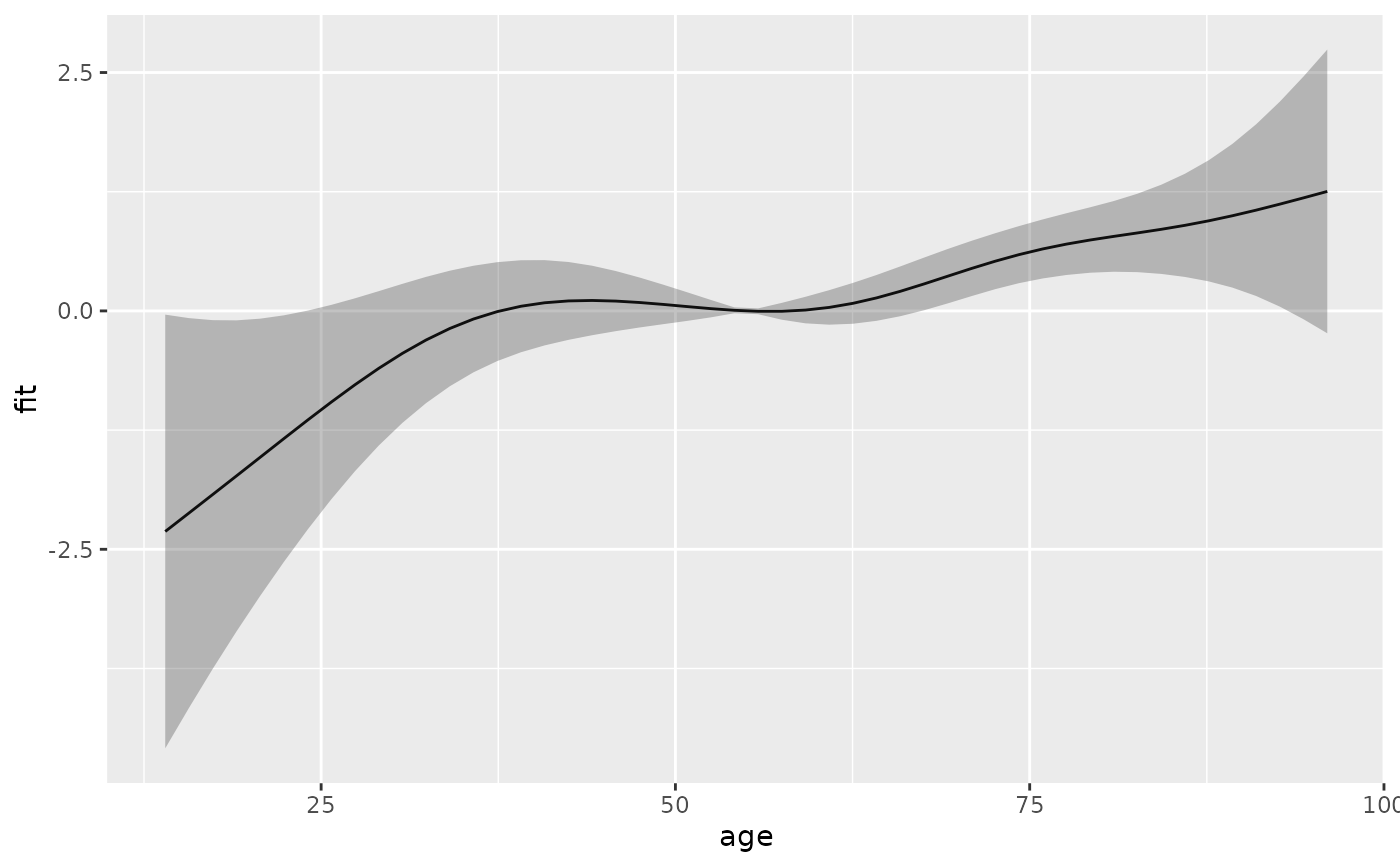Adds the contribution of a specific term to the linear predictor to the data specified by newdata. Essentially a wrapper to predict.gam, with type="terms". Thus most arguments and their documentation below is from predict.gam.

add_term(newdata, object, term, reference = NULL, ci = TRUE, se_mult = 2, ...)

## Arguments

newdata

A data frame or list containing the values of the model covariates at which predictions are required. If this is not provided then predictions corresponding to the original data are returned. If newdata is provided then it should contain all the variables needed for prediction: a warning is generated if not. See details for use with link{linear.functional.terms}.

object

a fitted gam object as produced by gam().

term

A character (vector) or regular expression indicating for which term(s) information should be extracted and added to data set.

reference

A data frame with number of rows equal to nrow(newdata) or one, or a named list with (partial) covariate specifications. See examples.

ci

logical. Indicates if confidence intervals should be calculated. Defaults to TRUE.

se_mult

The factor by which standard errors are multiplied to form confidence intervals.

...

Further arguments passed to predict.gam

## Examples

library(ggplot2)
ped <- as_ped(tumor, Surv(days, status)~ age, cut = seq(0, 2000, by = 100))
pam <- mgcv::gam(ped_status ~ s(tend) + s(age), family = poisson(),
offset = offset, data = ped)
#term contribution for sequence of ages
s_age <- ped %>% make_newdata(age = seq_range(age, 50)) %>%